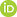Full Text:<2379>

Summary:<1455>

CLC number: O32

On-line Access: 2017-09-04

Revision Accepted: 2016-11-21

Crosschecked: 2017-08-15

Cited: 0

Clicked: 4159

Citations:  Bibtex RefMan EndNote GB/T7714ORCID:

Qiang-feng Lü

http://orcid.org/0000-0003-4750-7418

### -   Go to

Article info.
 Journal of Zhejiang University SCIENCE A 2017 Vol.18 No.9 P.704-717 http://doi.org/10.1631/jzus.A1600541Stochastic averaging of quasi partially integrable Hamiltonian systems under fractional Gaussian noise

 Author(s):  Qiang-feng Lü, Mao-lin Deng, Wei-qiu Zhu Affiliation(s):  Department of Mechanics, Zhejiang University, Hangzhou 310027, China; more Corresponding email(s):   wqzhu@zju.edu.cn Key Words:  Fractional Brownian motion (fBm), Fractional Gaussian noise (fGn), Quasi partially integrable Hamiltonian system, Stochastic averaging method, Stationary response Share this article to： More <<< Previous Article|Next Article >>>

Qiang-feng Lü, Mao-lin Deng, Wei-qiu Zhu. Stochastic averaging of quasi partially integrable Hamiltonian systems under fractional Gaussian noise[J]. Journal of Zhejiang University Science A, 2017, 18(9): 704-717.

@article{title="Stochastic averaging of quasi partially integrable Hamiltonian systems under fractional Gaussian noise",
author="Qiang-feng Lü, Mao-lin Deng, Wei-qiu Zhu",
journal="Journal of Zhejiang University Science A",
volume="18",
number="9",
pages="704-717",
year="2017",
publisher="Zhejiang University Press & Springer",
doi="10.1631/jzus.A1600541"
}

%0 Journal Article
%T Stochastic averaging of quasi partially integrable Hamiltonian systems under fractional Gaussian noise
%A Qiang-feng Lü
%A Mao-lin Deng
%A Wei-qiu Zhu
%J Journal of Zhejiang University SCIENCE A
%V 18
%N 9
%P 704-717
%@ 1673-565X
%D 2017
%I Zhejiang University Press & Springer
%DOI 10.1631/jzus.A1600541

TY - JOUR
T1 - Stochastic averaging of quasi partially integrable Hamiltonian systems under fractional Gaussian noise
A1 - Qiang-feng Lü
A1 - Mao-lin Deng
A1 - Wei-qiu Zhu
J0 - Journal of Zhejiang University Science A
VL - 18
IS - 9
SP - 704
EP - 717
%@ 1673-565X
Y1 - 2017
PB - Zhejiang University Press & Springer
ER -
DOI - 10.1631/jzus.A1600541

Abstract:
A stochastic averaging method for predicting the response of quasi partially integrable and non-resonant Hamiltonian systems to fractional Gaussian noise (fGn) with the Hurst index 1/2<H<1 is proposed. The averaged stochastic differential equations (SDEs) for the first integrals of the associated Hamiltonian system are derived. The dimension of averaged SDEs is less than that of the original system. The stationary probability density and statistics of the original system are obtained approximately from solving the averaged SDEs numerically. Two systems are worked out to illustrate the proposed stochastic averaging method. It is shown that the results obtained by using the proposed stochastic averaging method and those from digital simulation of original system agree well, and the computational time for the former results is less than that for the latter ones.

The traditional stochastic averaging method of quasi Hamiltonian system is based on the theory of diffusion process. An important property of diffusion process is the Markov property. For a long time, many researchers are wondering what the stochastic averaging method would be like if the system response processes is not Markov process. The fractional Brownian motion is known as a non-Markov process. And it is also known that the system response process have to be non-Markov process when the system is excited by fractional Gaussian noise. I value the subject discussed in this manuscript. It is the first time that the stochastic averaging method of quasi Hamiltonian system is developed to the case of non-Markov process.

### Reference

Biagini, F., Hu, Y., Øksendal, B., et al., 2008. Stochastic Calculus for Fractional Brownian Motion and Applications. Springer-Verlag, London, UK.Cheridito, P., Kawaguchi, H., Maejima, M., 2003. Fractional Ornstein-Uhlenbeck processes. Electronic Journal of Probability, 8:3.Deng, M.L., Zhu, W.Q., 2007. Stochastic averaging of MDOF quasi integrable Hamiltonian systems under wide-band random excitation. Journal of Sound and Vibration, 305(4-5):783-794.Deng, M.L., Zhu, W.Q., 2012. Stochastic energy transition of peptide bond under the action of hydrolytic enzyme. Probabilistic Engineering Mechanics, 27(1):8-13.Deng, M.L., Zhu, W.Q., 2016. Stochastic averaging of quasi non-integrable Hamiltonian systems under fractional Gaussian noise excitation. Nonlinear Dynamics, 83(1-2):1015-1027.Gu, W., Liu, Y., Hao, R., 2016. Attenuated model of pricing credit default swap under the fractional Brownian motion environment. Journal of Mathematical Finance, 06(02):64347.Huang, Z.L., Zhu, W.Q., Ni, Y.Q., et al., 2002. Stochastic averaging of strongly non-linear oscillators under bounded noise excitation. Journal of Sound and Vibration, 254(2):245-267.Jia, W.T., Zhu, W.Q., 2014. Stochastic averaging of quasi-partially integrable Hamiltonian systems under combined Gaussian and Poisson white noise excitations. Physica A: Statistical Mechanics and Its Applications, 398:125-144.Kaarakka, T., Salminen, P., 2011. On fractional Ornstein-Uhlenbeck processes. Communications on Stochastic Analysis, 7(1):121-133.

Kou, S.C., Xie, X.S., 2004. Generalized Langevin equation with fractional Gaussian noise: subdiffusion within a single protein molecule. Physical Review Letters, 93(18):180603.Li, F.G., Ai, B.Q., 2012. Fractional Gaussian noise-induced evolution and transition in anti-tumor model. The European Physical Journal B, 85(2):1-6.Mandelbrot, B.B., van Ness, J.W., 1968. Fractional Brownian motions, fractional noises and applications. SIAM Review, 10(4):422-437.Mishura, Y.S., 2008. Stochastic Calculus for Fractional Brownian Motion and Related Processes. Springer-Verlag, Berlin, Germany.Ni, X.H., Jiang, Z.Q., Zhou, W.X., 2009. Degree distributions of the visibility graphs mapped from fractional Brownian motions and multifractal random walks. Physics Letter A, 373(42):3822-3826.Papoulis, A., 1991. Probability, Random Variables and Stochastic Processes, 3rd Edition. McGraw-Hill, New York, USA.

Sithi, V.M., Lim, S.C., 1995. On the spectra of Riemann-Liouville fractional Brownian motion. Journal of Physics A: Mathematical and General, 28(11):2995-3003.Sliusarenko, O.Y., Gonchar, V.Y., Chechkin, A.V., et al., 2010. Kramers-like escape driven by fractional Gaussian noise. Physical Review E, 81(4):041119.Tabor, M., 1989. Chaos and Integrability in Nonlinear Dynamics: an Introduction. Wiley, New York, USA.

Xu, Y., Guo, R., Xu, W., 2014a. A limit theorem for the solutions of slow-fast systems with fractional Brownian motion. Theoretical and Applied Mechanics Letters, 4(1):013003.Xu, Y., Guo, R., Liu, D., et al., 2014b. Stochastic averaging principle for dynamical systems with fractional Brownian motion. Discrete and Continuous Dynamical Systems-Series B, 19(4):1197-1212.Zeng, Y., Zhu, W.Q., 2011. Stochastic averaging of quasi-nonintegrable-Hamiltonian systems under Poisson white noise excitation. Journal of Applied Mechanics, 78(2):021002.Zhu, W.Q., Huang, Z.L., Yang, Y.Q., 1997. Stochastic averaging of quasi-integrable Hamiltonian systems. Journal of Applied Mechanics, 64(4):975-984.Zhu, W.Q., Huang, Z.L., Suzuki, Y., 2002. Stochastic averaging and Lyapunov exponent of quasi partially integrable Hamiltonian systems. International Journal of Non-Linear Mechanics, 37(3):419-437.Zunino, L., Pérez, D.G., Martín, M.T., et al., 2008. Permutation entropy of fractional Brownian motion and fractional Gaussian noise. Physics Letter A, 372(27-28):4768-4774.